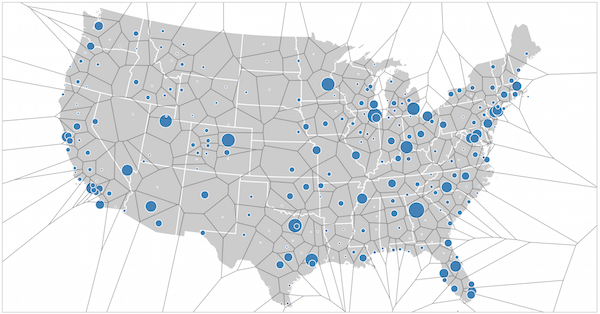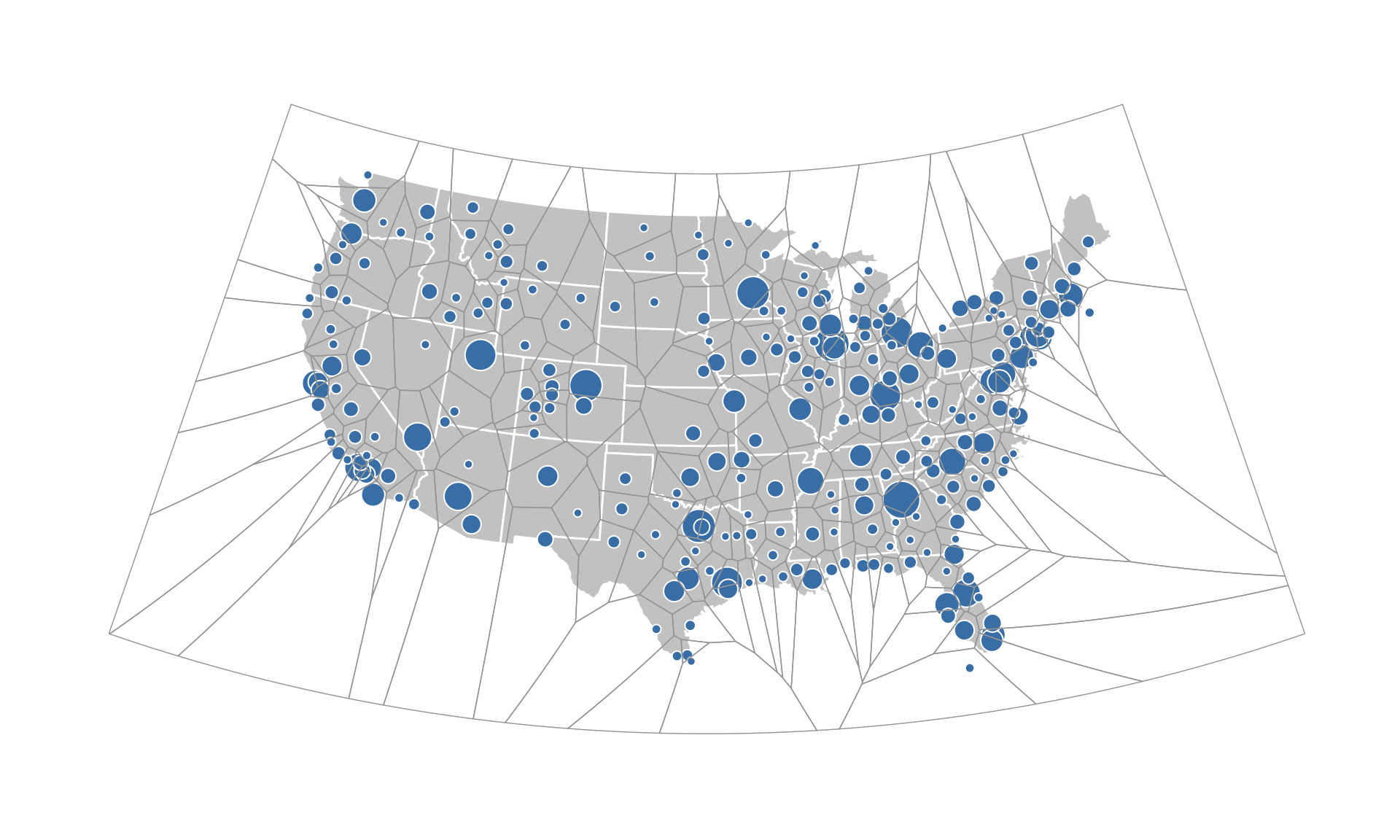# Making Static/Interactive Voronoi Map Layers In ggplot/leaflet

Despite having shown various ways to overcome D3 cartographic envy, there are always more examples that can cause the green monster to rear it’s ugly head.

Take the Voronoi Arc Map example.For those in need of a primer, a Voronoi tesslation/diagram is:

a partitioning of a plane into regions based on distance to points in a specific subset of the plane. That set of points (called seeds, sites, or generators) is specified beforehand, and for each seed there is a corresponding region consisting of all points closer to that seed than to any other. Wikipedia

We can overlay a Voronoi tessalation on top of a map in R as well thanks to the `deldir` package (which has been around since the “S” days!). Let’s get (most of) the package requirements cruft out of the way, first:

```library(sp) library(rgdal) library(deldir) library(dplyr) library(ggplot2) library(ggthemes)```

Now we’ll [ab]use the data from the Arc Map example:

```flights <- read.csv("http://bl.ocks.org/mbostock/raw/7608400/flights.csv", stringsAsFactors=FALSE) airports <- read.csv("http://bl.ocks.org/mbostock/raw/7608400/airports.csv", stringsAsFactors=FALSE)```

Since the D3 example cheats and only uses the continental US (CONUS) we’ll do the same and we’ll also filter out only those airports mentioned in the `flights` data and get the total # of incoming/outgoing flights for each airport:

```conus <- state.abb[!(state.abb %in% c("AK", "HI"))] airports <- filter(airports, state %in% conus, iata %in% union(flights\$origin, flights\$destination)) orig <- select(count(flights, origin), iata=origin, n1=n) dest <- select(count(flights, destination), iata=destination, n2=n) airports <- left_join(airports, select(mutate(left_join(orig, dest), tot=n1+n2), iata, tot)) %>% filter(!is.na(tot))```

Since we’re going to initially plot polygons in ggplot (and, eventually, in leaflet), we’ll need to work with `Spatial` objects, so let’s make those airport lat/lon pairs into a `SpatialPointsDataFrame`:

```vor_pts <- SpatialPointsDataFrame(cbind(airports\$longitude, airports\$latitude), airports, match.ID=TRUE)```

The `deldir` function returns a pretty complex object. Thankfully, the authors of the package realized that one might just want the polygons from the computation and pre-made a function: `tile.list` for computing/extracting them. Those polygons aren’t, however, closed and we really want to keep the airport data associatd with them, so we need to close the polygons and associate the data. Since we’re likely going to repeat this task, let’s make it a (very badly named) function:

```SPointsDF_to_voronoi_SPolysDF <- function(sp) {   # tile.list extracts the polygon data from the deldir computation vor_desc <- tile.list(deldir(sp@coords[,1], sp@coords[,2]))   lapply(1:(length(vor_desc)), function(i) {   # tile.list gets us the points for the polygons but we # still have to close them, hence the need for the rbind tmp <- cbind(vor_desc[[i]]\$x, vor_desc[[i]]\$y) tmp <- rbind(tmp, tmp[1,])   # now we can make the Polygon(s) Polygons(list(Polygon(tmp)), ID=i)   }) -> vor_polygons   # hopefully the caller passed in good metadata! sp_dat <- sp@data   # this way the IDs _should_ match up w/the data & voronoi polys rownames(sp_dat) <- sapply(slot(SpatialPolygons(vor_polygons), 'polygons'), slot, 'ID')   SpatialPolygonsDataFrame(SpatialPolygons(vor_polygons), data=sp_dat)   }```

Before we can make the plots, we need to put the `Spatial` objects into the proper form for ggplot2 (and get the U.S. state map):

```vor <- SPointsDF_to_voronoi_SPolysDF(vor_pts)   vor_df <- fortify(vor)   states <- map_data("state")```

Now we can have some fun. Let’s try to mimic the D3 example map as closely as possible. We’ll lay down the CONUS map, add a points layer for the the airports, sizing & styling them just like the D3 example. Note that we order the points so that the smallest ones appear on top (so we can still see them).

We’ll then lay down our newly created Voronoi layer. We’ll also use the same projection (Albers) that the D3 examples uses:

```gg <- ggplot() # base map gg <- gg + geom_map(data=states, map=states, aes(x=long, y=lat, map_id=region), color="white", fill="#cccccc", size=0.5) # airports layer gg <- gg + geom_point(data=arrange(airports, desc(tot)), aes(x=longitude, y=latitude, size=sqrt(tot)), shape=21, color="white", fill="steelblue") # voronoi layer gg <- gg + geom_map(data=vor_df, map=vor_df, aes(x=long, y=lat, map_id=id), color="#a5a5a5", fill="#FFFFFF00", size=0.25) gg <- gg + scale_size(range=c(2, 9)) gg <- gg + coord_map("albers", lat0=30, lat1=40) gg <- gg + theme_map() gg <- gg + theme(legend.position="none") gg```While that’s pretty, it’s not exactly useful. I’m sure there are times when it’s important to show the Voronoi polygons, but they are especially useful when they are used to help with user interface interactions.

In the case of this map, some airport “bubbles” are very small and many overlap, making a “click” (or even “hover”) a potentially painstaking task for someone looking to get more data out of the visualization. The D3 example uses Voronoi polygons to make it super-easy for the user to hover over a map area and get more info about the flights for the closest airport to the mouse pointer.

We’ll use the leaflet htmlwidget to do something similar. Until I can figure out “hover” events for R+leaflet, you’ll have to live with “click”.

First we’ll need some additional packages:

```library(leaflet) library(rgeos) library(htmltools)```

And, we’ll also need a U.S. shapefile (which we simplify since the polygons are pretty detailed and that’s not necessary for this vis):

```url <- "http://eric.clst.org/wupl/Stuff/gz_2010_us_040_00_500k.json" fil <- "gz_2010_us_040_00_500k.json"   if (!file.exists(fil)) download.file(url, fil, cacheOK=TRUE)   states_m <- readOGR("gz_2010_us_040_00_500k.json", "OGRGeoJSON", verbose=FALSE) states_m <- subset(states_m, !NAME %in% c("Alaska", "Hawaii", "Puerto Rico")) dat <- states_m@data # gSimplify whacks the data bits states_m <- SpatialPolygonsDataFrame(gSimplify(states_m, 0.05, topologyPreserve=TRUE), dat, FALSE)```

The leaflet vis idiom is similar to the ggplot idiom. I’m using a base tile layer since I was too lazy to figure out how to change the leaflet default gray background map color. The map polygons are added, then the circles/bubbles (note that you work in meters with `addCircles` which lets leaflet scale the bubbles as you zoom in/out). Finally, the Voronoi layer is added. I kept the stroke visible purely for demonstration purposes. You need to keep `fill=TRUE` otherwise the Voronoi layer won’t get click/hover events and once I figure out how to trigger popups on hover and use a static popup layer, this will let users hover around the map to get the underlying airport flight information.

```leaflet(width=900, height=650) %>% # base map addProviderTiles("Hydda.Base") %>% addPolygons(data=states_m, stroke=TRUE, color="white", weight=1, opacity=1, fill=TRUE, fillColor="#cccccc", smoothFactor=0.5) %>% # airports layer addCircles(data=arrange(airports, desc(tot)), lng=~longitude, lat=~latitude, radius=~sqrt(tot)*5000, # size is in m for addCircles O_o color="white", weight=1, opacity=1, fillColor="steelblue", fillOpacity=1) %>% # voronoi (click) layer addPolygons(data=vor, stroke=TRUE, color="#a5a5a5", weight=0.25, fill=TRUE, fillOpacity = 0.0, smoothFactor=0.5, popup=sprintf("Total In/Out: %s", as.character(vor@data\$tot)))```

I made the Voronoi layer very light, so you may want to keep it there as a cue for the user. How you work with it is completely up to you.

Now you have one less reason to be envious of the D3 cartographers!Amazon Author Page

## 4 Comments →Making Static/Interactive Voronoi Map Layers In ggplot/leaflet

1. Pingback: Distilled News | Data Analytics & R

2.Joshua

What a nice script! Thanks for sharing ;)

3.Alex ponce

I my god…. you are an alchemist!!! how beautiful & clever code….

mil mil mil gracias

Alex

This site uses Akismet to reduce spam. Learn how your comment data is processed.